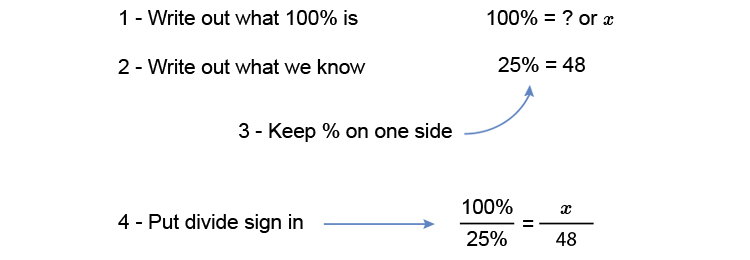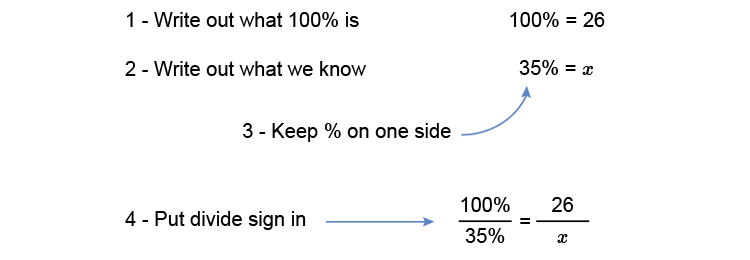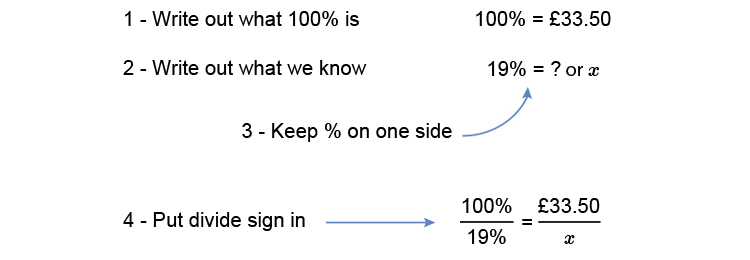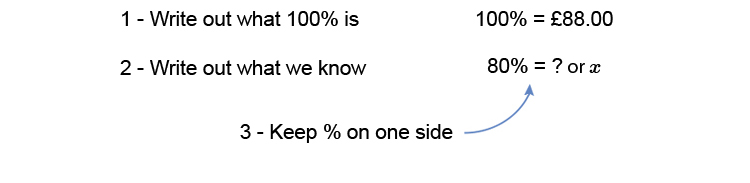# Finding a number

Example 1

48 is 25% of what number?Multiply both sides by 48 to get x on its own (what ever you do to one side you do to the other).

(4\ \ cancel100times48)/cancel25=(xtimescancel48)/cancel48

4times48=x

x=4times48

x=192

Answer: 48 is 25% of 192

Example 2

What is 35% of 26?Multiply both sides by x to get x on its own (what ever you do to one side you do to the other).

(xtimes100)/35=(26timescancelx)/cancelx

(100x)/35=26

Multiply both sides by 35 to get x on its own.

(100xtimescancel35)/cancel35=26times35

100x=26times35

Divide both sides by 100 to get x on its own

(cancel100x)/cancel100=(26times35)/100

x=(26times35)/100

x=9.1

Answer: 9.1 is 35% of 26

Example 3

I’m going to buy £33.50, excluding tax, worth of food. The sales tax is 19%. How much tax do I pay and how much will the total bill be?

This is the same as saying:

What is 19% of £33.50?Multiply both sides by x to get x on its own (remember what ever you do to one side you do to the other).

(100timesx)/19=(33.50timescancelx)/cancelx

(100timesx)/19=33.50

Multiply both sides by 19 to get x on its own

(100xtimescancel19)/cancel19=33.50times19

100x=33.50times19

Divide both sides by 100 to get X on its own

(cancel100x)/cancel100=(33.50times19)/100

x=(33.50times19)/100

x=6.37

Answer: The tax is £6.37 and the total price will therefore be £6.37 + £33.50 = £39.87

Example 4

A coat costs £88.00 and is then discounted in a sale by 20%. How much will the sale price be?NOTE:

With a discount of 20% the new price is 80% of the original.Multiply both sides by x to get x on its own (what you do to one side you do to the other).

(100timesx)/80=(88.00timescancelx)/cancelx

(100x)/80=88.00

Multiply both sides by 80 to get x on its own.

(100xtimescancel80)/cancel80=88.00times80

100x=88.00times80

Divide both sides by 100 to get x on its own.

(cancel100x)/cancel100=(88.00times80)/100

x=(88.00times80)/100

x=70.40

Answer: The sale price will be £70.40